# Almost-split sequence

(diff) ← Older revision | Latest revision (diff) | Newer revision → (diff)

Auslander–Reiten sequence

Roughly speaking, almost-split sequences are minimal non-split short exact sequences. They were introduced by M. Auslander and I. Reiten in 1974–1975 and have become a central tool in the theory of representations of finite-dimensional algebras (cf. also Representation of an associative algebra).

Letbe an Artin algebra, i.e.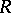is an associative ring with unity that is finitely generated as a module over its centre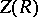, which is a commutative Artinian ring.

Let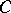be an indecomposable non-projective finitely-generated left-module. Then there exists a short exact sequence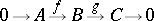(a1)

in, the category of finitely-generated left-modules, with the following properties:

i)andare indecomposable;

ii) the sequence does not split, i.e. there is no section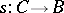of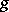(a homomorphism such that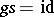), or, equivalently, there is no retraction of(a homomorphismsuch that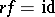);

iii) given anywith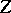indecomposable andnot an isomorphism, there is a lift ofto(i.e. a homomorphism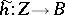insuch that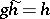);

iv) given anywithindecomposable andnot an isomorphism, there is a homomorphism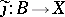such that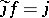.

Note that if iii) (or, equivalently, iv)) were to hold for all, not just thosethat are not isomorphisms, the sequence (a1) would be split, whence "almost split" . Moreover, a sequence (a1) with these properties is uniquely determined (up to isomorphism) by, and also by. This is the basic Auslander–Reiten theorem on almost-split sequences, [a1], [a8], [a9], [a10], [a11].

For convenience (things also work more generally), let nowbe a finite-dimensional algebra over an algebraically closed field. The category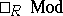is a Krull–Schmidt category (Krull–Remak–Schmidt category), i.e. a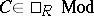is indecomposable if and only if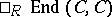, the endomorphism ring of, is a local ring and (hence) the decomposition of a module ininto indecomposables is unique up to isomorphism.

Letbe an indecomposable and consider the contravariant functor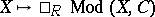. The morphismsthat do not admit a section (i.e. an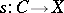such that) form a vector subspace. Let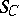be the quotient functor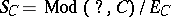. Then, for an indecomposable,ifis isomorphic toand zero otherwise. Sois a simple functor. (All functors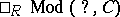,,are viewed as-functors, i.e. functors that take their values in the category of vector-spaces.) Ifis indecomposable, then (the Auslander–Reiten theorem, [a4], p.4) the simple functoradmits a minimal projective resolution of the form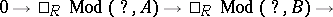Ifis projective,is zero, otherwiseis indecomposable.

Ifis not projective, the sequence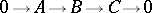is exact and is the almost-split sequence determined by.

This functorial definition is used in [a5] in the somewhat more general setting of exact categories.

For a good introduction to the use of almost-split sequences, see [a6]; see also [a3], [a5] for comprehensive treatments. See also Riedtmann classification for the use of almost-split sequences and the Auslander–Reiten quiver in the classification of self-injective algebras.

The Bautista–Brunner theorem says that ifis of finite representation type andis an almost-split sequence, thenhas at most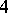terms in its decomposition into indecomposables; also, if there are indeed, then one of these is projective-injective. This can be generalized, [a7].

How to Cite This Entry:
Almost-split sequence. Encyclopedia of Mathematics. URL: http://encyclopediaofmath.org/index.php?title=Almost-split_sequence&oldid=12003
This article was adapted from an original article by M. Hazewinkel (originator), which appeared in Encyclopedia of Mathematics - ISBN 1402006098. See original article